# probabilities module 3

Problem Set 3.1

1. According to the National Oceanic and Atmospheric Administration (NOAA), between 1851 and 2013 there were 290 hurricanes that hit the U.S. Coast. Of these, 117 were Category 1 hurricanes, 76 were Category 2 hurricanes, 76 were Category 3 hurricanes, 18 were Category 4 hurricanes, and 3 were Category 5 hurricanes.
Make a probability distribution for this data. If a hurricane hits the U.S. coast, what is the probability that the hurricane will be a Category 1 hurricane.

2. In a certain high school, 54% of the students are female, 46% of the students are male. If you select a student at random from this school, what is the probability that the student will be a female? What is the probability that the student will be a male?

3. A bag contains jelly beans of various colors. In the bag, 26% of the jelly beans are red, 20% are black, 35% are green, and 19% are yellow. If you choose a jelly bean at random from the bag, what is the probability that the jelly bean will be black? What is the probability that it will be yellow?

4. Find the answer to each of the following by first reducing the fractions as much as possible:

a) P(17,8)=

b) C(19,15)=

5. Find the answer to each of the following by first reducing the fractions as much as possible:

a) P(670,3)=

b) C(883,879)=

6. Suppose you are going to make a password that consists of 5 characters chosen from {2,3,6,7,a,c,k,n,p}. How many different passwords can you make if you cannot use any character more than once in each password?

7. Suppose that 10 countries submit bids for the summer Olympics. The Olympic committee will select 4 finalists out of these 10 countries. In how many ways can the Olympic committee pick these 10 finalists?

8. Suppose that you must choose three people to meet with a customer. You have nine people to choose from. In how many different ways can you choose these three people?

9. There are three openings in an accounting firm: one opening is in personal accounts, another is in corporate accounts, and another is in international accounts. There are 12 candidates available for these three jobs (any of the 12 candidates can fill any of the three jobs). In how many ways can these three openings be filled?

# Problem Set 3.2

Problem Set 3.2

1. Suppose A and B are two events with probabilities:Find the following:a)

b)

c)

2. Suppose A and B are two events with probabilities:Find the following:a)

b)

c)

3. Suppose A and B are two events with probabilities: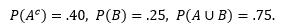Find the following:a)

b)

c)

d)

4. Suppose A and B are two events with probabilities: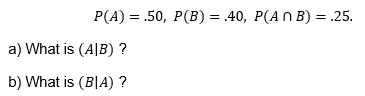a)

b)

5. Suppose A and B are two events with probabilities: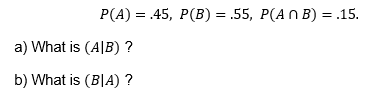a)

b)

6. Suppose A and B are two events with probabilities:a)

b)

7. Suppose A and B are two events with probabilities: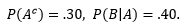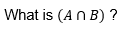a)

b)

8. The probability that a certain type of light bulb will last 650 hours or more is .75. The probability that the light bulb will last 800 hours or more is .30. Suppose that the bulb is 650 hours old and is still working, what is the probability that the bulb will last at least 800 hours?

9. Suppose that in a certain type of washing machine that the probability that a belt will fail is .03 and the probability that the agitator will fail is .01. The probability that the belt and the agitator will fail simultaneously is .008. Suppose that you discover that the belt in a washing machine failed, what is the probability that the agitator has also failed?

# Problem Set 3.3

Problem Set 3.3

1. During the last year, Labrador Retriever Dogs were produced in a city by three breeders:

57 % from Breeder A; 18 % from Breeder B; 25 % from Breeder C.

It has been found that 7.5 % of the dogs from Breeder A, 12 % of the dogs from Breeder B, and 40 % of the dogs from Breeder C have a specific genetic defect. Suppose you get one of these dogs and find that the dog has this specific genetic defect, what is the probability the dog came from Breeder C?